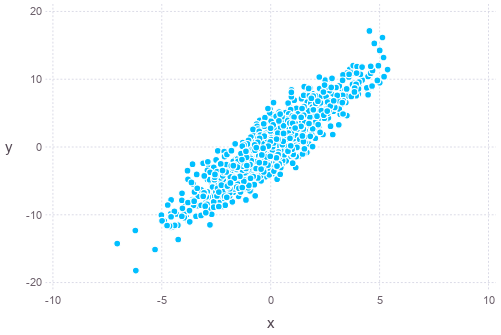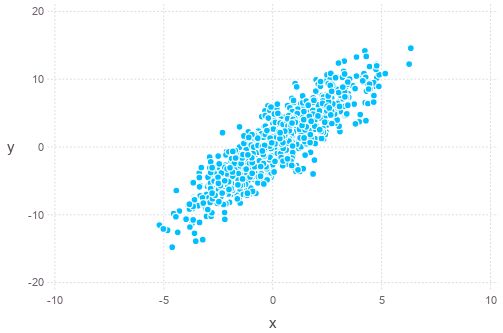Multivariate normal distribution has the following p.d.f.:

$f(\vec{x}) = \frac{1}{\sqrt{(2\pi)^n|\mathbf{\Sigma}|}} \exp(-\frac{1}{2}(\vec{x}-\vec{\mu})^T\mathbf{\Sigma}^{-1}(\vec{x}-\vec{\mu}))$

and for a bivariate in specific, it is:

$f(x_1,x_2) = \frac{1}{2\pi\sqrt{\sigma_1^2\sigma_2^2-\sigma_{12}^2}} \exp\left(-\frac{ \sigma_2^2(x_1-\mu_1)^2 - 2\sigma_{12}(x_1-\mu_1)(x_2-\mu_2) + \sigma_1^2(x_2-\mu_2)^2 }{ 2(\sigma_1^2\sigma_2^2-\sigma_{12}^2) } \right)$

where the covariance $$\sigma_{12}^2 = \rho\sigma_1\sigma_2$$. See also the note on multivariate Gaussian distribution.

The multivariate normal random variable can be considered as a combination of the mean vector and zero-mean multivariate random variable. Assume $$\mathbf{y}$$ to be a $$n$$-vector of iid standard normal random variables, i.e., $$\bar{\mathbf{y}}=\mathbf{0}$$ and $$E[\mathbf{yy}^T]=\mathbf{I}$$. Let there be a matrix of constants $$\mathbf{S}$$ of size $$m\times n$$, and a $$m$$-vector of constants (the mean) $$\mathbf{m}$$, and define

$\mathbf{x} = \mathbf{Sy} + \mathbf{m}$

Then $$\mathbf{x}$$ is a multivariate normal random variable, which the covariance matrix is

\begin{align} \Sigma_{\mathbf{x}} &= E[(\mathbf{x}-\mathbf{m})(\mathbf{x}-\mathbf{m})^T] \\ &= E[(\mathbf{Sy})(\mathbf{Sy})^T] \\ &= E[\mathbf{Sy}\mathbf{y}^T\mathbf{S}^T] \\ &= \mathbf{S}E[\mathbf{yy}^T]\mathbf{S}^T \\ &= \mathbf{SS}^T \end{align}

Finding $$\mathbf{S} = \Sigma_{\mathbf{x}}^{1/2}$$ given covariance matrix $$\Sigma_{\mathbf{x}}$$ is typically using Cholesky decomposition.

Taking again bivariate as an example. If the covariance matrix is

$\Sigma_{\mathbf{x}} = \begin{bmatrix}3 & 2 \\ 2 & 5\end{bmatrix}$

In Julia, we can plot a set of samples of the distribution as follows (assumed zero-mean):

Sigma = [4 9; 9 25]
S = chol(Sigma) # upper triangular part, i.e. S' * S == Sigma
iidnorm = randn(2,1000)
mvnorm = S' * iidnorm
plot(x=mvnorm[1,:], y=mvnorm[2,:], Geom.point)Alternatively, such sampling can also be done using Monte Carlo method, such as the Gibbs sampling. The algorithm is Gibbs sampling is as follows:

• Assume some sensible value for the multivariate random variable $$x^{(0)} = (x_1^{(0)}, x_2^{(0)}, \cdots, x_n^{(0)})$$ as initial value
• For $$k=0$$ up to $$k=M+N$$
• For $$i=1$$ up to $$i=n$$, i.e. each component of the multivariate random variable
• Set $$x_i^{(k+1)}$$ according to probability $$p(x_i\mid x_1^{(k+1)}, \cdots, x_{i-1}^{(k+1)}, x_{i+1}^{(k)}, \cdots, x_n^{(k)})$$
• Discard the first $$M$$ samples of $$x^{(k)}$$ above and use only the last $$N$$ as terminal distribution

For the particular case of zero-mean bivariate normal random variables, the loop above means:

• For $$k=0$$ up to $$k=M$$
• set $$x_1^{(k+1)} = \rho x_2^{(k)}\sigma_1/\sigma_2 + \sqrt{\sigma_1^2(1-\rho^2)} Z_1$$ where $$Z_1 \sim N(0,1)$$
• set $$x_2^{(k+1)} = \rho x_1^{(k+1)}\sigma_2/\sigma_1 + \sqrt{\sigma_2^2(1-\rho^2)} Z_2$$ where $$Z_2 \sim N(0,1)$$

The formula $$x_1^{(k+1)} = \rho x_2^{(k)} + \sqrt{\sigma_1^2(1-\rho^2)} Z_1$$ comes from the following:

\begin{align} p(x_1 \mid x_2) &= \frac{p(x_1, x_2)}{p(x_2)} \\ &= \frac{ \frac{1}{2\pi\sigma_1\sigma_2\sqrt{1-\rho^2}} \exp\left( -\frac{x_1^2/\sigma_1^2 - 2\rho x_1x_2/(\sigma_1\sigma_2)+x_2^2/\sigma_2^2}{2(1-\rho^2)} \right) }{ \frac{1}{\sqrt{2\pi\sigma_2^2}} \exp\left(-\frac{x_2^2}{2\sigma^2}\right) } \\ &= \frac{1}{\sqrt{2\pi}\sigma_1\sqrt{1-\rho^2}} \exp\left( -\frac{(x_1/\sigma_1 - \rho x_2/\sigma_2)^2}{2(1-\rho^2)} \right) \\ &= \frac{1}{\sqrt{2\pi}\sigma_1\sqrt{1-\rho^2}} \exp\left( -\frac{(x_1 - \rho x_2\sigma_1/\sigma_2)^2}{2\sigma_1^2(1-\rho^2)} \right) \\ &= N(\rho x_2\sigma_1/\sigma_2, \sigma_1^2(1-\rho^2)) \end{align}

And this is the corresponding code:

Sigma = [4 9; 9 25]
sigma1 = sqrt(Sigma[1,1])
sigma2 = sqrt(Sigma[2,2])
rho = Sigma[1,2]/(sigma1*sigma2)
states = []
push!(states, [0,0])
for i = 1:1500
Z1,Z2 = randn(2)
x1, x2 = last(states)
x1 = rho * x2 * sigma1 / sigma2 + sqrt(sigma1^2 * (1-rho^2)) * Z1
x2 = rho * x1 * sigma2 / sigma1 + sqrt(sigma2^2 * (1-rho^2)) * Z2
push!(states, [x1,x2])
end
states = hcat(states...)
plot(x=states[1,501:end], y=states[2,501:end], Geom.point)